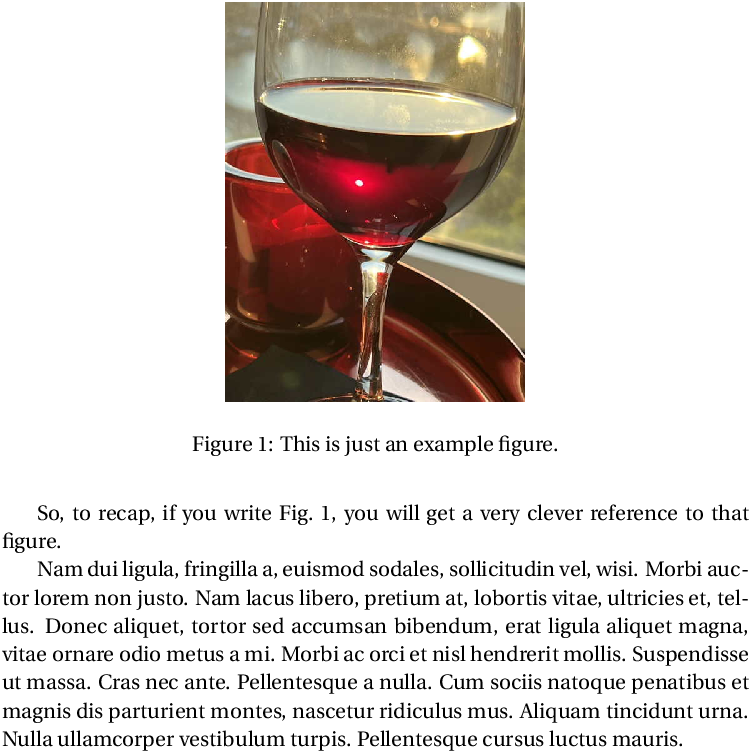# The cleveref Package

Documentation figures , references A package for making cross-referencing much easier. Very useful with hyperref. \usepackage{cleveref} % make cross-referencing much easier

Suppose that you have a figure labeled with the command \label{fig:sample}, such as by using

\begin{figure}[t!]
\centering
\includegraphics[width=2in]{sample}
\caption{This is just an example figure.}
\label{fig:sample}
\end{figure}


In "normal" LaTeX, you create a cross reference to this figure with a command such as

Fig.~\ref{fig:gamma}


which would output something like Fig. 3. When you use the hyperref package to turn cross references into active links, only the number 3 would be part of the clickable link.

The cleveref package improves this system by (a) handling the Fig.~ part for you. If you load cleveref with options nameinlink and capitalize, it will include the Fig.~ part in the clickable link. If you prefer to have things like equations and figures referenced with lowercase (e.g. eq. (3) instead of Eq. (3), don't use the option capitalize.

So, to recap, if you write \cref{fig:sample}, you will get a
very clever reference to that figure.

\lipsum


## Defining your own reference types

Suppose you define your own environment with its corresponding counter. For example, a problem environment with associated \newcounter{problem}. Inside the problem environment, you define the label with a statement like

\label[problem]{prob:<thelabel>}


Then you can get cleveref to work with problems by putting

\crefname{problem}{Problem}{Problems}